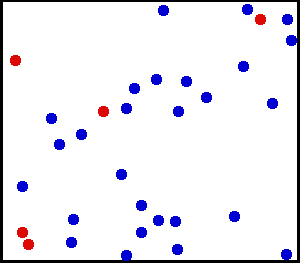• ANTLR4 是一个非常厉害的程序/库，可以用来生成 Lexer 和 Parser，而且生成的接口非常易用。昨天匆匆把 The Definitive ANTLR 4 Reference 扫了一遍，现在把一些常用的东西记下来。

• 最近在看SICP，然后看到练习2.6，十分的有趣，刚好和上学期计科导讲过的自然数的构造有点类似。题目首先给了0和加一的实现，然后问1和2应该怎么直接定义，再问如何直接定义加法。

``````(define zero (lambda (f) (lambda (x) x)))
(define (add-1 n)
(lambda (f) (lambda (x) (f ((n f) x)))))
``````
• 之前物理课的时候看到了PPT上一张分子热运动的图，觉得很有趣，于是那个星期就做了个二维球体完全弹性碰撞的简单物理引擎。非常凑巧的是，刚好这个东西可以拿来做最后的物理大作业，也算是非常开心吧。先放上这次物理大作业做的三个热学演示吧，其实核心代码是一样的，只不过根据不同演示的需要做了一定的修改：

写引擎之前我隐约知道，好像大部分的物理引擎都是在每一帧里面，让每个物体往前前进一点，然后当两个物体重叠之后再做碰撞的处理。但是我对这一套理论十分的不了解，而且总是觉得这么做十分的麻烦，又怕当碰撞十分复杂的时候（比如接连有好多个物体碰撞）会出问题，所以我决定按照另外一个十分显然的思路来写引擎。

• ## 问题的引入

最近有若干同学和我讨论在实现 C++ `vector` 容器的时候如何实例化一个没有默认构造函数的类。比如下面的代码：

``````#include <cstddef>

template <class T>
class vector {
size_t capacity, size;
T *data;
public:
vector() :
capacity(10), // for example
size(0),
data(new T[capacity]) {
}

~vector() {
delete [] data;
}
};

struct Point {
int x, y;
Point(int a, int b): x(a), y(b) {}
};

int main() {
vector<int> a;
vector<Point> b;
}

``````

在实例化 `vector<int>` 的时候不会有问题，然而当实例化 `vector<Point>` 的时候就会出错了

• 因为物理实验作图的原因，我有幸接触了 `matplotlib` ，配合 `scipy` 的其他组件，可以完成我所有需要的任务（我的需求都是非常初级的 :-) ）。鉴于接下来可能转战 `R` ，所以特地把最近 `matplotlib` 的使用经验总结一下。

## import

``````import numpy as np
from pylab import *
from scipy.optimize import leastsq
from matplotlib.font_manager import FontManager
``````

## 简单的绘图

``````# 折线图：
plot(x, y)
plot(x, y, '-')
plot(x, y, '-', linewidth=2)

# 散点图
plot(x, y, 'o', markersize=2)
plot(x, y, ',')
``````